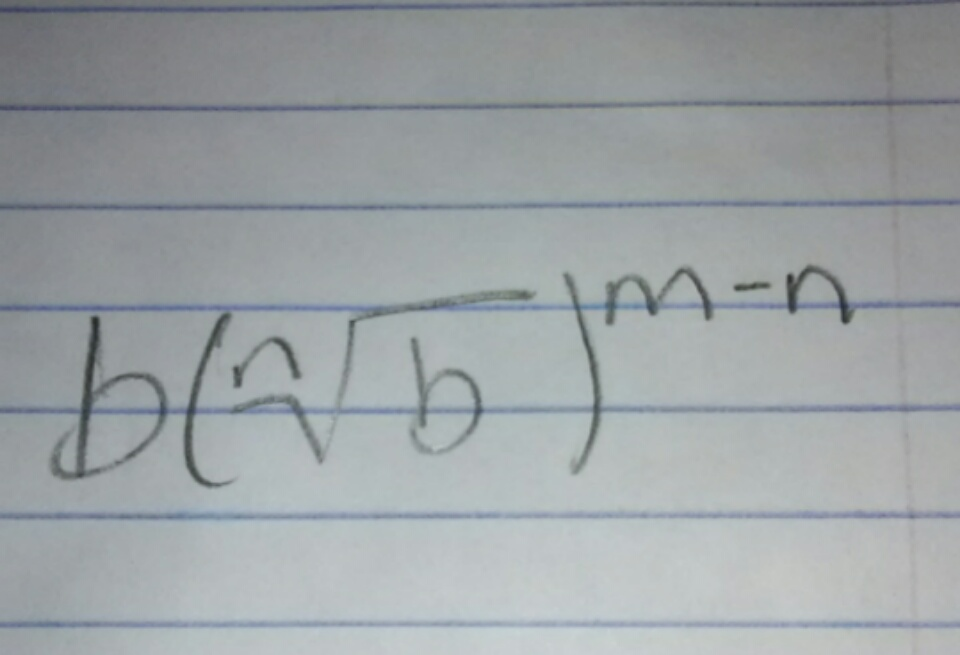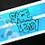# Easier Formula for Rational Exponents?

During my Math II class today, I was looking at yesterdays homework for Rational Exponents and how to simplify them, 16 to the 5/2 power, etc. I was looking through it and got curious and began to simplify a problem, 8 to the 5/3 power, 32 of course, however while I was doing it I got curious and started working backwards, through this I reached a simpler version of the problem being 8([3rd root of 8] to the 5-3 power) [Looks simpler on paper]. I then reverted this to the base formula from b to the m/n power to be b([nth root of b] to the m-n power). In this form you can plug in any base combined with the index and the base's m and turn it into a problem which only needs to be simplified. I am curious if anyone has thought of this yet and if so, where does it originate. I did this with various numbers -1, 1, 2, 3 (simple ones) and saw that it worked with all of them. Let me know if anyone has seen this before please!Note by Sage Derpy
3 years, 5 months ago

This discussion board is a place to discuss our Daily Challenges and the math and science related to those challenges. Explanations are more than just a solution — they should explain the steps and thinking strategies that you used to obtain the solution. Comments should further the discussion of math and science.

When posting on Brilliant:

• Use the emojis to react to an explanation, whether you're congratulating a job well done , or just really confused .
• Ask specific questions about the challenge or the steps in somebody's explanation. Well-posed questions can add a lot to the discussion, but posting "I don't understand!" doesn't help anyone.
• Try to contribute something new to the discussion, whether it is an extension, generalization or other idea related to the challenge.

MarkdownAppears as
*italics* or _italics_ italics
**bold** or __bold__ bold
- bulleted- list
• bulleted
• list
1. numbered2. list
1. numbered
2. list
Note: you must add a full line of space before and after lists for them to show up correctly
paragraph 1paragraph 2

paragraph 1

paragraph 2

[example link](https://brilliant.org)example link
> This is a quote
This is a quote
    # I indented these lines
# 4 spaces, and now they show
# up as a code block.

print "hello world"
# I indented these lines
# 4 spaces, and now they show
# up as a code block.

print "hello world"
MathAppears as
Remember to wrap math in $$ ... $$ or $ ... $ to ensure proper formatting.
2 \times 3 $2 \times 3$
2^{34} $2^{34}$
a_{i-1} $a_{i-1}$
\frac{2}{3} $\frac{2}{3}$
\sqrt{2} $\sqrt{2}$
\sum_{i=1}^3 $\sum_{i=1}^3$
\sin \theta $\sin \theta$
\boxed{123} $\boxed{123}$